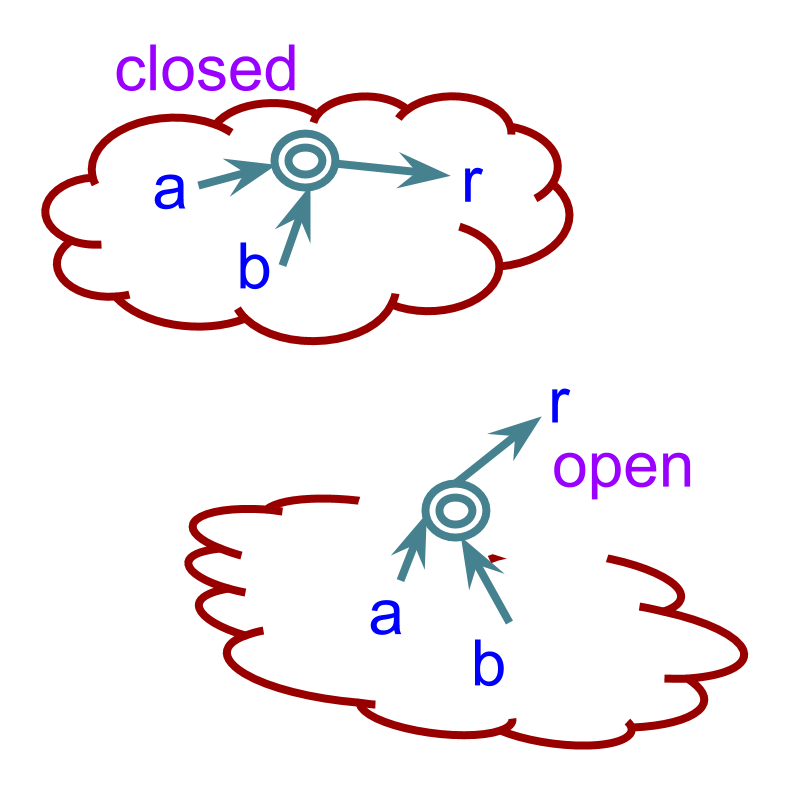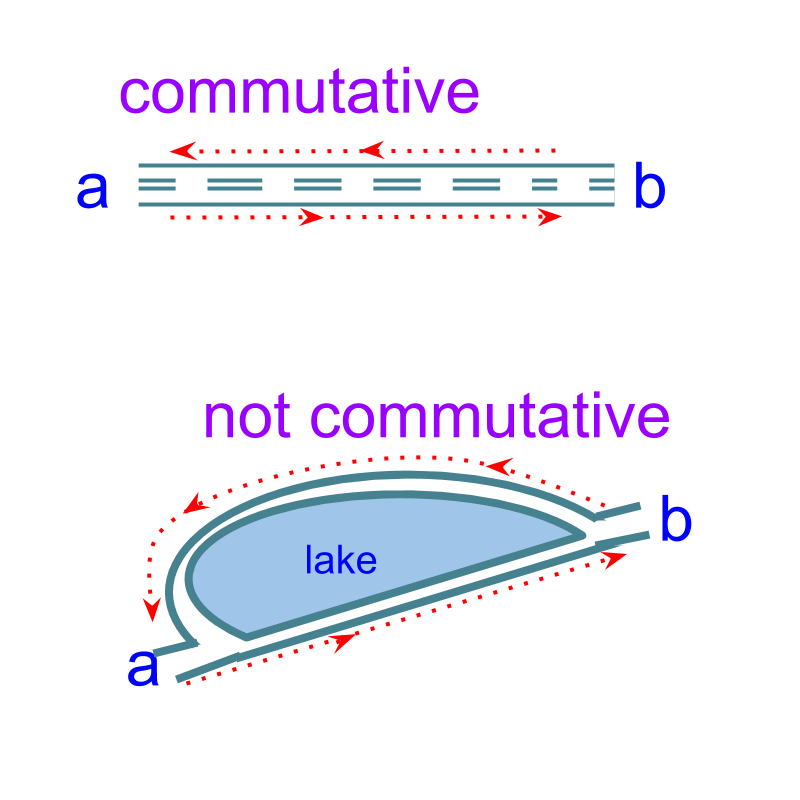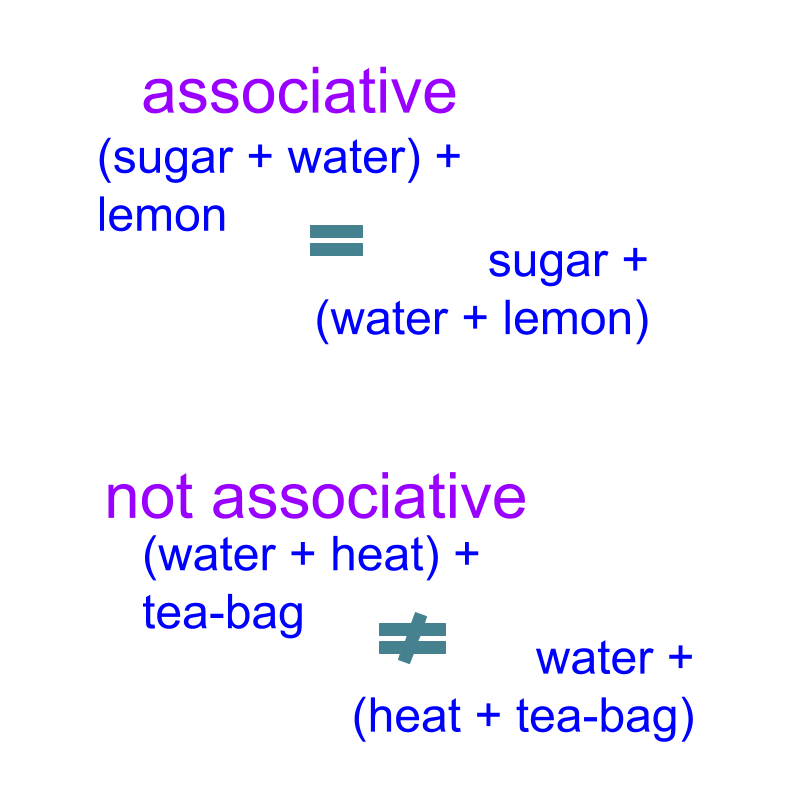maths > algebra

Laws and Properties of Arithmetics : Addition

what you'll learn...

Overview

»  Closure Property
→  if $x,y\in ℝ$$x , y \in \mathbb{R}$, then $x+y\in ℝ$$x + y \in \mathbb{R}$

»  Commutative Property
→  $x+y=y+x$$x + y = y + x$

»  Associative Property
→  $\left(x+y\right)+z=x+\left(y+z\right)$$\left(x + y\right) + z = x + \left(y + z\right)$

→  $0\in ℝ$$0 \in \mathbb{R}$, such that $x+0=x$$x + 0 = x$

→  $-x\in ℝ$$- x \in \mathbb{R}$ for any $x\in ℝ$$x \in \mathbb{R}$ such that $x+\left(-x\right)=0$$x + \left(- x\right) = 0$

»  Subtraction is to be handled as additive inverse for the properties
this is important as algebra extensively involves these properties
→  Commutative property involving subtraction : $x-y$$x - y$ is given as $x+\left(-y\right)=\left(-y\right)+x$$x + \left(- y\right) = \left(- y\right) + x$
→  Associative property involving subtraction : $\left(x-y\right)-z$$\left(x - y\right) - z$ is given as $\left(x+\left(-y\right)\right)+\left(-z\right)=x+\left(\left(-y\right)+\left(-z\right)\right)$$\left(x + \left(- y\right)\right) + \left(- z\right) = x + \left(\left(- y\right) + \left(- z\right)\right)$

closed means within

Consider the numbers $2$$2$ and $3$$3$. Is $2+3$$2 + 3$ a real number? "yes, a real number".

That is, for any real numbers $p,q$$p , q$, $p+q$$p + q$ is always a real number

"closure" means closed and not openClosure Property of Addition: Given $p,q\in ℝ$$p , q \in \mathbb{R}$, $p+q\in ℝ$$p + q \in \mathbb{R}$.

Closure Property applied to Subtraction: Given $p,q\in ℝ$$p , q \in \mathbb{R}$. $p-q\in ℝ$$p - q \in \mathbb{R}$.

Proof:
Given $p,q\in ℝ$$p , q \in \mathbb{R}$
$-q\in ℝ$$- q \in \mathbb{R}$ as per Additive Inverse Property
$p+\left(-q\right)\in ℝ$$p + \left(- q\right) \in \mathbb{R}$ as per Closure property of Addition
$⇒p-q\in ℝ$$\implies p - q \in \mathbb{R}$

Using Closure Property: Given $p,q,r,s\in ℝ$$p , q , r , s \in \mathbb{R}$, $p+q-r+s$$p + q - r + s$, the subexpression $p+q-r$$p + q - r$ is a real number and can be considered as a single number for any other property.

For example as per commutative property $p+q-r+s=s+p+q-r$$p + q - r + s = s + p + q - r$, in which $p+q-r$$p + q - r$ is considered to be a single real number.

Consider the numbers $2$$2$ and $3$$3$. Which of the following is true?
$2+3=3+2$$2 + 3 = 3 + 2$ or
$3+2$$3 + 2$ does not equal to $2+3$$2 + 3$

The answer is "$2+3=3+2$$2 + 3 = 3 + 2$".

Given $p,q\in ℝ$$p , q \in \mathbb{R}$; $p+q=q+p$$p + q = q + p$

forward and backward

The word "commute" means "to go to and fro between two places on a regular basis".Commutative Property of Addition: Given $p,q\in ℝ$$p , q \in \mathbb{R}$, $p+q=q+p$$p + q = q + p$.

Commutative Property applied to Subtraction: $p-q=-q+p$$p - q = - q + p$.
Note: Subtraction has to be handled as inverse of addition, $p-q=p+\left(-q\right)$$p - q = p + \left(- q\right)$ and then commutative property can be used.

Using Commutative Property: Given $p,q,r\in ℝ$$p , q , r \in \mathbb{R}$, the expression $p+q+p-q+r-p+2r$$p + q + p - q + r - p + 2 r$ is simplified to $p+3r$$p + 3 r$. students may work this out to understand.

with this or that

Given $p,q,r\in ℝ$$p , q , r \in \mathbb{R}$. The expression $\left(p+q\right)+r$$\left(p + q\right) + r$ equals $p+\left(q+r\right)$$p + \left(q + r\right)$

In the first expression, $q$$q$ is added first with $p$$p$ and then $r$$r$ is added to the result.
In the second expression $q$$q$ is added first with $r$$r$ and then $p$$p$ is added to the result.
Either way, the result is same.

For example, $\left(2+3\right)+7=2+\left(3+7\right)$$\left(2 + 3\right) + 7 = 2 + \left(3 + 7\right)$

The word "associate" means 'to connect with; to join'.Associative Property of Addition: Given $p,q,r\in ℝ$$p , q , r \in \mathbb{R}$. $\left(p+q\right)+r=p+\left(q+r\right)$$\left(p + q\right) + r = p + \left(q + r\right)$.

Associative Property applied to subtraction: $\left(p-q\right)-r=p+\left(-q-r\right)$$\left(p - q\right) - r = p + \left(- q - r\right)$.
Note: Subtraction has to be handled as inverse of addition, $\left(p-q\right)-r=\left(p+\left(-q\right)\right)+\left(-r\right)$$\left(p - q\right) - r = \left(p + \left(- q\right)\right) + \left(- r\right)$ and then associative property can be used.

zero

Given $p\in ℝ$$p \in \mathbb{R}$. What is $p+0=p$$p + 0 = p$

Additive Identity Property: For any $p\in ℝ$$p \in \mathbb{R}$, there exists $0\in ℝ$$0 \in \mathbb{R}$ such that $p+0=p$$p + 0 = p$. Additive Identity applied to Subtraction: $p-0=p$$p - 0 = p$

Note: $-0=0$$- 0 = 0$ and so $p-0=p+\left(-0\right)=p+0=p$$p - 0 = p + \left(- 0\right) = p + 0 = p$.

inverse

Given $p\in ℝ$$p \in \mathbb{R}$. What is $p-p=0$$p - p = 0$

Additive Inverse Property: For any $p\in ℝ$$p \in \mathbb{R}$, there exists $-p\in ℝ$$- p \in \mathbb{R}$ such that $p+\left(-p\right)=0$$p + \left(- p\right) = 0$.

summary

The properties together are named as CADI properties of addition. The abbreviation CADI is a simplified form of the first letters of Closure, Commutative, Associative, Distributive, Inverse, and Identity properties.

Note: Distributive property is shared with multiplication and is explained in the next page.

•  Closure Property
if $x,y\in ℝ$$x , y \in \mathbb{R}$, then $x+y\in ℝ$$x + y \in \mathbb{R}$

•  Commutative Property
$x+y=y+x$$x + y = y + x$

•  Associative Property
$\left(x+y\right)+z=x+\left(y+z\right)$$\left(x + y\right) + z = x + \left(y + z\right)$

$0\in ℝ$$0 \in \mathbb{R}$, such that $x+0=x$$x + 0 = x$

$-x\in ℝ$$- x \in \mathbb{R}$ for any $x\in ℝ$$x \in \mathbb{R}$ such that $x+\left(-x\right)=0$$x + \left(- x\right) = 0$

Subtraction is to be handled as additive inverse and properties of addition applies to subtraction in the form of addition.

This is important as algebra extensively uses these properties.

→  Commutative property involving subtraction : $x-y$$x - y$ is given as $x+\left(-y\right)$$x + \left(- y\right)$$=\left(-y\right)+x$$= \left(- y\right) + x$
→  Associative property involving subtraction : $\left(x-y\right)-z$$\left(x - y\right) - z$ is given as $\left(x+\left(-y\right)\right)+\left(-z\right)$$\left(x + \left(- y\right)\right) + \left(- z\right)$$=x+\left(\left(-y\right)+\left(-z\right)\right)$$= x + \left(\left(- y\right) + \left(- z\right)\right)$

Outline

The outline of material to learn "Algebra Foundation" is as follows.

→   Numerical Arithmetics

→   Arithmetic Operations and Precedence

→   Properties of Comparison

→   Properties of Multiplication

→   Properties of Exponents

→   Algebraic Expressions

→   Algebraic Equations

→   Algebraic Identities

→   Algebraic Inequations